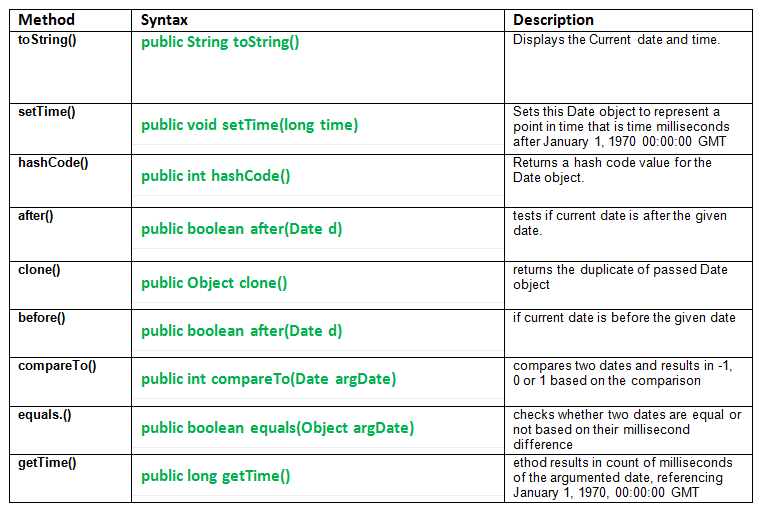Open In App

# util.date class methods in Java with ExamplesFollowing are some important date class methods :

1. .toString() : java.util.Date.tostring() method is a java.util.Date class method.It displays the Current date and time.
Here Date object is converted to a string and represented as:

` day mon dd hh:mm:ss zz yyyy `

day : day of the week
mon : month
dd : day of the month
hh : hour
mm : minute
ss : second
zz : time zone
yyyy : year upto 4 decimal places

```Syntax:
public String toString()
Return:
a string representation of the given date.
```
2. .setTime() : java.util.Date.setTime() method is a java.util.Date class method. Sets this Date object to represent a point in time that is time milliseconds after January 1, 1970 00:00:00 GMT.
```Syntax:
public void setTime(long time)
Parameters:
time : the number of milliseconds.
```
3. .hashCode() : java.util.Date.hashCode() method is a java.util.Date class method. Returns a hash code value for the Date object. The result is exclusive OR of the two halves of the primitive long value returned by the getTime() method.
```Syntax:
public int hashCode()
Return:
a hash code value for the Date object.
```

Java Code to illustrate the use of .toString(), setTime(), hashCode() methods.

 `// Java Program explaining util.date class methods//``// use of .toString(), setTime(), hashCode() methods``import` `java.util.*;  ``// class having access to Date class methods`` ` `public` `class` `NewClass``{``    ``public` `static` `void` `main(String[] args)``    ``{``        ``Date mydate = ``new` `Date();`` ` `        ``// Displaying the current date and time``        ``System.out.println(``"System date : "``+ mydate.toString() );`` ` `        ``// Is used to set time by milliseconds. Adds 15680 ``        ``// milliseconds to January 1, 1970 to get new time.``        ``mydate.setTime(``15680``);`` ` `        ``System.out.println(``"Time after setting:  "` `+ mydate.toString());`` ` `        ``int` `d = mydate.hashCode();``        ``System.out.println(``"Amount (in ms) by which time"` `+ ``                           ``" is shifted :  "` `+ d);``    ``}``}`

Output of Java code:

```System date : Tue Nov 01 02:37:18 IST 2016
Time after setting:  Thu Jan 01 05:30:15 IST 1970
Amount (in milliseconds)  by which time is shifted :  15680
```
4. .after() : java.util.Date.after() method tests if current date is after the given date.
```Syntax:
public boolean after(Date d)
Parameters:
d : date
Return:
true if and only if the instant represented by this Date object is strictly later
than the instant represented by 'when'; else false
Exception:
NullPointerException - if Date object is null.
```
5. .clone() : java.util.Date.clone() method returns the duplicate of passed Date object.
```Syntax:
public Object clone()
Return:
a clone of this instance.
```
6. .before() : java.util.Date.after() method tests if current date is before the given date.
```Syntax:
public boolean before(Date d)
Parameters:
d : date
Return:
true if and only if the instant represented by this Date object is strictly earlier
than the instant represented by 'when'; else false
Exception:
NullPointerException - if when is null.
```
7. Java Code to illustrate the use of after(), clone(), before() methods.

 `// JAVA program explaining Date class methods``// after(), clone(), before()``import` `java.util.Date;``public` `class` `NewClass``{``    ``public` `static` `void` `main(String[] args)``    ``{``        ``// create 2 dates``        ``Date date1 = ``new` `Date(``2016``, ``11``, ``18``);``        ``Date date2 = ``new` `Date(``1997``, ``10``, ``27``);`` ` `        ``// Use of after() to check date2 is after date1``        ``boolean` `a = date2.after(date1);``        ``System.out.println(``"Is date2 is after date1 : "` `+ a);`` ` `        ``// Use of after() to check date2 is after date1``        ``a = date1.after(date2);``        ``System.out.println(``"Is date1 is after date2 : "` `+ a);``        ``System.out.println(``""``);`` ` `        ``// Use of clone() method``        ``Object date3 = date1.clone();``        ``System.out.println(``"Cloned date3 :"` `+ date3.toString());``        ``System.out.println(``""``);`` ` `        ``// Use of before() to check date2 is after date1``        ``boolean` `b = date2.before(date1);``        ``System.out.println(``"Is date2 is before date1 : "` `+ a);``    ``}``}`

Output :

```Is date2 is after date1 : false
Is date1 is after date2 : true

Cloned date3 :Mon Dec 18 00:00:00 IST 3916

Is date2 is before date1 : true
```
8. .compareTo() : java.util.Date.compareTo() method compares two dates and results in -1, 0 or 1 based on the comparison.
```Syntax:
public int compareTo(Date argDate)
Parameters:
argDate : another date to compare with
Result:
0  : if the argumented date = given date.
-1 : if the argumented date > given date.
1  : if the argumented date < given date.
```
9. .equals() : java.util.Date.equals() method checks whether two dates are equal or not based on their millisecond difference.
```Syntax:
public boolean equals(Object argDate)
Parameters:
argDate : another date to compare with
Result:
true if both the date are equal; else false.
```
10. .getTime() : java.util.Date.getTime() method results in count of milliseconds of the argumented date, referencing January 1, 1970, 00:00:00 GMT.
```Syntax:
public long getTime()
Result:
milliseconds of the argumented date, referencing January 1, 1970, 00:00:00 GMT.
```
11. Java Code to illustrate the use of compareTo(), getTime(), equals() methods.

 `// Java program explaining Date class methods``// compareTo(), getTime(), equals()``import` `java.util.*;``public` `class` `NewClass``{``    ``public` `static` `void` `main(String[] args)``    ``{``        ``Date d1 = ``new` `Date(``97``, ``10``, ``27``);``        ``Date d2 = ``new` `Date(``97``, ``6``, ``12``);`` ` `        ``// Use of compareto() method``        ``int` `comparison = d1.compareTo(d2);    ``// d1 > d2``        ``int` `comparison2 = d2.compareTo(d1);   ``// d2 > d1``        ``int` `comparison3 = d1.compareTo(d1);   ``// d1 = d1`` ` `        ``System.out.println(``"d1 > d2 : "` `+ comparison);``        ``System.out.println(``"d1 < d2 : "` `+ comparison2);``        ``System.out.println(``"d1 = d1 : "` `+ comparison3);``        ``System.out.println(``""``);`` ` `        ``// Use of equal() method``        ``boolean` `r1 = d1.equals(d2);``        ``System.out.println(``"Result of equal() r1 : "` `+ r1);`` ` `        ``boolean` `r2 = d1.equals(d1);``        ``System.out.println(``"Result of equal() r2 : "` `+ r2);``        ``System.out.println(``""``);`` ` ` ` `        ``// Use of getTime() method``        ``long` `count1 = d1.getTime();``        ``long` `count2 = d1.getTime();``        ``System.out.println(``"Milliseconds of d1 : "` `+ count1);``        ``System.out.println(``"Milliseconds of d2 : "` `+ count2);``    ``}``}`

Output :

```d1 > d2 : 1
d1 < d2 : -1
d1 = d1 : 0

Result of equal() r1 : false
Result of equal() r2 : true

Milliseconds of d1 : 880569000000
Milliseconds of d2 : 880569000000
```

This article is contributed by Mohit Gupta. If you like GeeksforGeeks and would like to contribute, you can also write an article using write.geeksforgeeks.org or mail your article to review-team@geeksforgeeks.org. See your article appearing on the GeeksforGeeks main page and help other Geeks.